Subsections

## 3.4.2 Empirical Models for LON

Empirical Models for LON started with the initial attempt to understand the physical mechanisms by Gordon et al. . Teuschler et al. ,  in the same year suggested that the height of the generated nanodot is proportional to the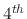root of the pulse time, (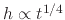). Although this empirical power law was a good fit to the experimental data, there was no relation to the traditional idea of oxide growth models, such as those mentioned in Chapter 2. The first model based on the physical interactions during LON was introduced in 1997 by Stievenard et al. . After analyses were done with thick oxides , it was found that a logarithmic time dependence ofversusis a good fit to the available experimental data withranging from 0.01 to 1000s andup to 50nm. More refined models for ICM-AFM and NCM-AFM lithographies were later suggested by Snow et al.  and Calleja et al. , respectively. The models are for relatively thin oxides and pulse voltages below 30V, while for oxides grown under very high voltage (30 to 50V), the Faradic current, which is present in the water meniscus, turns to ohmic current, and an additional ring of oxide is grown along the outside of the nanodot . These structures, grown under very high voltage, are beyond the scope of the models presented in this work, but similar methods may be used in the future in order to include such features in LON simulations.

Figure 3.11: Modeling approach for a hemispherical AFM needle tip versus a rough AFM needle tip. (a) Dot charge used to model AFM with a hemispherical needle tip and (b) Ring of charges used to model AFM with a rough needle tip.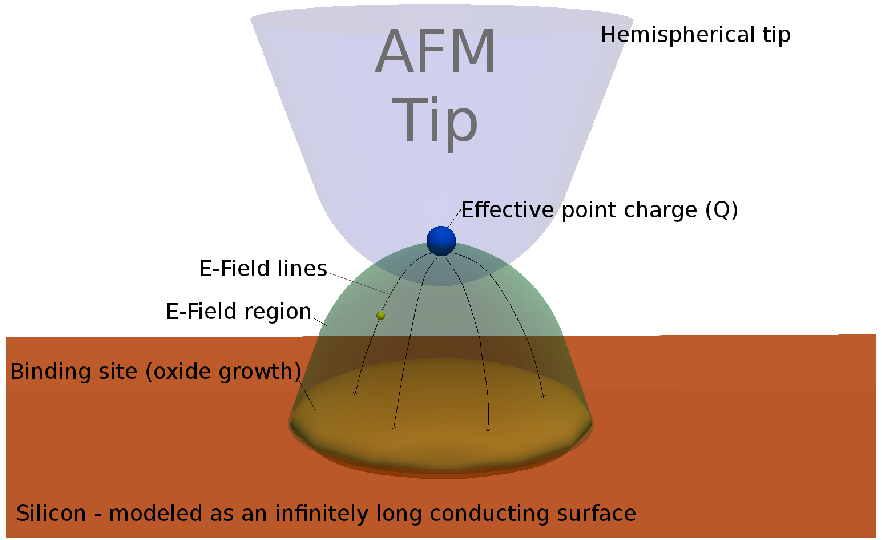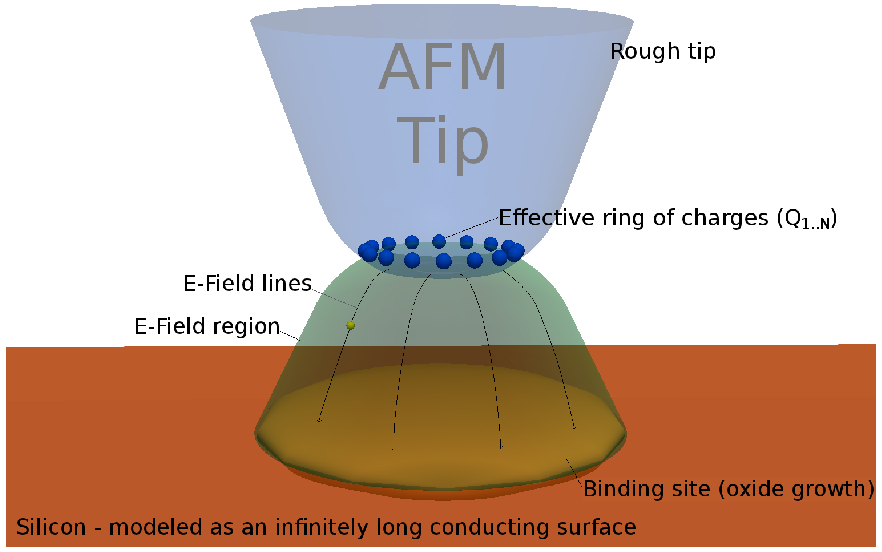(a) Dot charge. (b) Ring of charges.

Three types of AFM tip shapes have been analyzed in the literature . The different shapes are for a rough, hemispherical, and blunt tip configuration, which can be modeled using a ring charge, point charge, and ring charge, respectively. We present two models: one for a hemispherical tip shape, which involves only one charged dot and one for all other tip shapes, where multiple charged dots are required in order to model the needle tip. The schematics of this approach are shown in Figure 3.11a and Figure 3.11b, valid for a hemispherical and blunt needle tip, respectively. For the implemented model, it is assumed that all oxyanions are generated at the effective point source of the AFM needle tip. This simplifies the model, while not having a significant consequence on the model's accuracy . The oxyanions traverse through the water meniscus along electric field lines, finally colliding with the sample surface, whose evolution is visualized using the LS method , .

Using the method of image charges, the voltage and electric field strength in the water meniscus region has been calculated , . The effects of surrounding ions on the electric field strength and recombination reactions between ions to form water were neglected . Mesa et al.  suggest that each AFM needle can be represented as a series of charged particles distributed along the structure of the needle. The presented model implements this idea with the use of point charges, which is valid for all types of AFM needles.

### 3.4.2.1 Surface Charge Density Distribution for a Hemispherical Needle Tip

As discussed in the previous section, the model representing the shape of a hemispherical AFM generated nanodot follows the Surface Charge Density (SCD) distribution, which is derived by replacing the AFM needle tip with an effective point source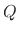and the silicon substrate surface by an infinitely long conducting plane. The image charge method is then applied to find the voltage at every location in the water meniscus region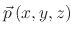: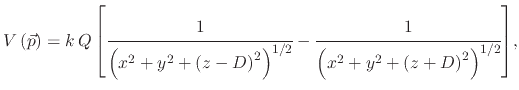(105)

where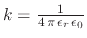,is the effective point charge at a distancefrom the surface, and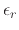is the relative permittivity of water. Given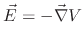, the electric field in each direction can be found: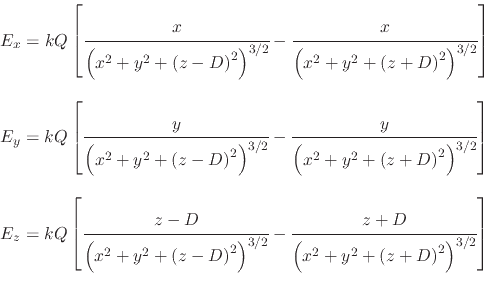(106)

The induced SCD on the surface is represented as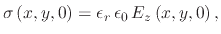(107)(108)

A model has been developed which utilizes (3.37) in order to generate a topographical representation of an AFM generated nanodot. This model implements a MC rejection technique, or accept-reject algorithm, to build the nanodot, which is very time and memory expensive, when large aspect ratio problems are required. Having an explicit particle distribution is preferred.

### 3.4.2.2 Surface Charge Density Distribution for a Rough Needle Tip

As mentioned in Section 3.4, in order to simulate the nanodot growth, initiated using a rough AFM tip, the needle may be modeled as a ring of charges at a given height above the silicon surface. The ring of charges is modeled by a desired number of dot charges surrounding the AFM tip circumference. When multiple dot charges are used to represent the AFM needle, the equation for the surface charge density becomes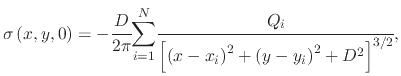(109)

where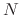is the total number of charged dots,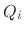and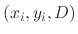are the effective charge and the location of the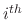dot, respectively. The maximum possible SCD distribution occurs, when all the charges are concentrated at a single point, making the charged circle's radius zero: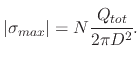(110)

With (3.38), simulations of AFM needle tips are not limited to those represented with a ring of charges. Rather, they can include charges which are located at any position, allowing it to properly mimic the behavior of the applied needle. The equation may also be used to study the effects of placing a second needle nearby. Performing multiple AFM oxidations in parallel with an array of needles is a popular way by which LON can have higher throughput and faster processing times.

### 3.4.2.3 Model for scanning tunneling microscope lithography

There are not many models for LON with a scanning tunneling microscope. The reason for this is that, quickly after the discovery of STM nano-oxidation, the AFM was introduced and shown to be a better tool for LON. The limitations of STM lithography when compared to AFM lithography are higher tip degradation due to reduced conductivity, difficulty in evaluating the exact lithography after printing due to the STM's inability to distinguish SiO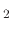from the ambient, slow dip velocity required for stable operation, and the requirement for STM to be operated in UHV . An analysis of STM-induced oxidation was performed by Kramer et al. , , which indicated that oxidation is induced by the electrical field between the tip and silicon sample and not by the energetic electrons from the tip directly. With decreasing Relative Air Humidity (RAH), the generated oxide also decreases in size, down to a threshold humidity of 10%, beyond which, no oxidation takes place . Increasing the applied reverse bias voltage also increases the oxide size, in both height and width. However, it is shown that the inverse oxide line width has a logarithmic influence from the applied bias, as seen in Figure 3.12, which is a reproduction of Figure 3 from .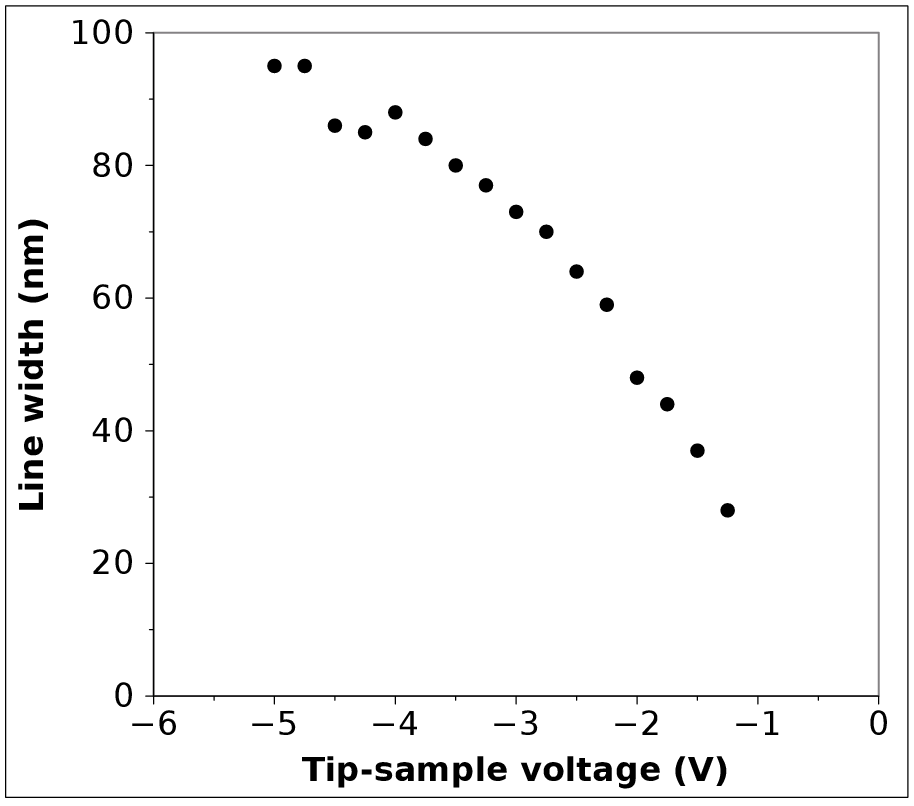### 3.4.2.4 Model for contact mode lithography

The first physical-based model for CM nano-oxidation with an AFM was suggested by Stievenard et al. . The model is based on the Cabrera and Mott model and it suggests a linear relation between the oxide height () and bias voltage (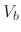) and a logarithmic relationship between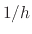and,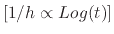. Another model, which shows the same patterns as those presented in  is shown in . Both models assumed a static RAH, with Stievenard's model setting it to 70%. This is ignored as a parameter; however, it is well known that the amount of water which is available during oxidation influences the growth rate and size of the oxide pattern .

From the initial assumption that the oxidation reaction is similar to the Cabrera and Mott model, but adapted for very thin oxide films, a model is developed in :

If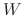is the energy which an ion must overcome to diffuse, the kinetics of growth can then be given by: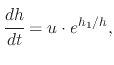(111)

where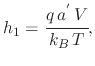(112)

with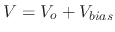,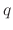is the electronic charge of an electron,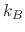is the Boltzmann constant,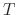is the temperature,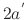is the barrier width between two interstitial sites, and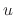is given by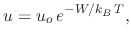(113)

with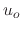in the order of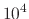cm/s . The kinetics of the oxide width are not included in the model, because they depend strongly on the AFM needle tip and, therefore, on the generated water meniscus.

### 3.4.2.5 Model for intermittent contact mode lithography

In order to simulate oxidation patterns using an AFM operating in ICM, the model presented in  is implemented. The equation which governs the height of the grown oxide is given by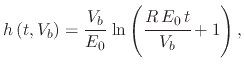(114)

whereis the pulse time,is the bias voltage, and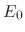and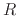are fitting parameters, with=45V/nm and=1.5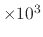nm/s. At high voltages and long pulse times, the height deviates significantly due to the formation of a water bridge between the tip and the wafer . The simulator is adjusted to handle this behavior by increasingto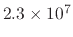nm/s and introducing a series voltage drop . Figure 3.13 shows the measured and modeled heights for this process.

The simulator also requires a dependence relationship for the Full Width at Half Maximum (FWHM) of the grown oxide, which is required in order to be able to generate a complete oxide nanodot. The work presented in  provides some measured results, shown in Figure 3.14. An empirical equation for the FWHM developed to fit the measured results is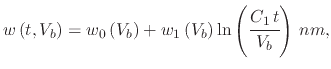(115)

where(116)

whereis given in seconds,in volts, and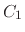,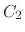,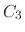, and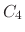are fitted values,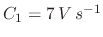,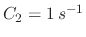,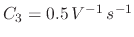, and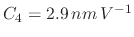The developed model suggests that the width of the profile has a logarithmic dependence on the pulse time and inverse logarithmic dependence on the applied voltage, similar to the dependence observed from the oxide height model. Once again, the model had to be adjusted for the case, when high voltages and long pulse times are applied.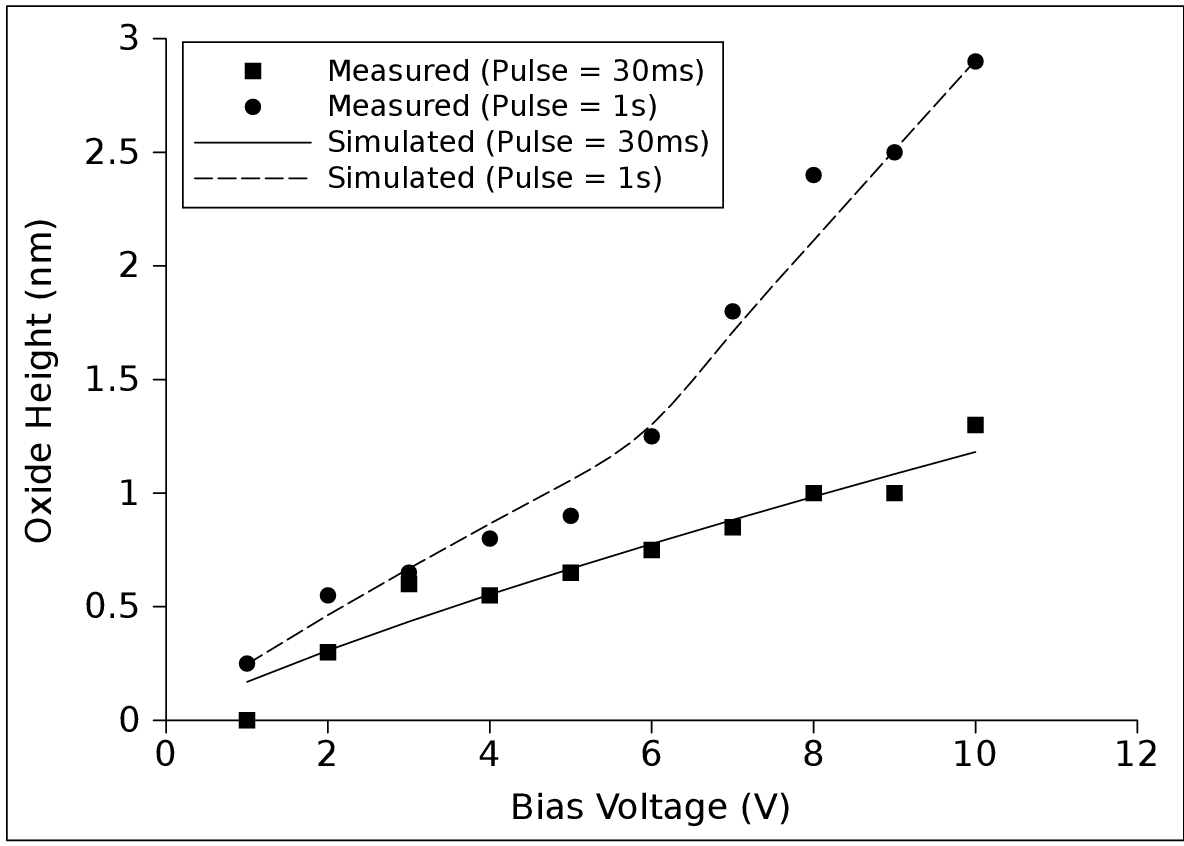### 3.4.2.6 Model for nanodots generated in NCM

When operating the AFM in NCM mode, the formation of a field-induced liquid bridge is required in order to provide oxyanions (OH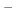, O), used to form the oxide. The liquid bridge also limits the lateral extensions of the region to be oxidized. The model, implemented in the process simulator, is described in , where it is suggested that the width and height of a produced pattern have a linear dependence on the applied bias voltage, while a logarithmic dependence exists for the pulse duration.

The empirical equation which governs the height of the oxide dot, produced using NCM, and presented in  is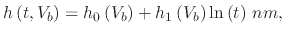(117)

where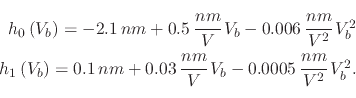(118)

Similarly, the equation which governs the width of the oxide dot, represented as the FWHM is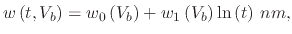(119)

where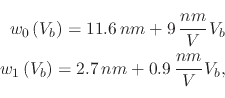(120)

and size, voltage, and time are expressed in nanometers, volts, and seconds, respectively. The dependence behavior of the oxide height and width with respect to the bias voltage and pulse time is evident from Figure 3.15, where (3.46) and (3.48) are represented graphically. The linear relationship between voltage and nanodot size (height or width) at a single pulse time value is evident, while the logarithmic relationship between the pulse time and nanodot size (height or width) at a single voltage level is also visualized.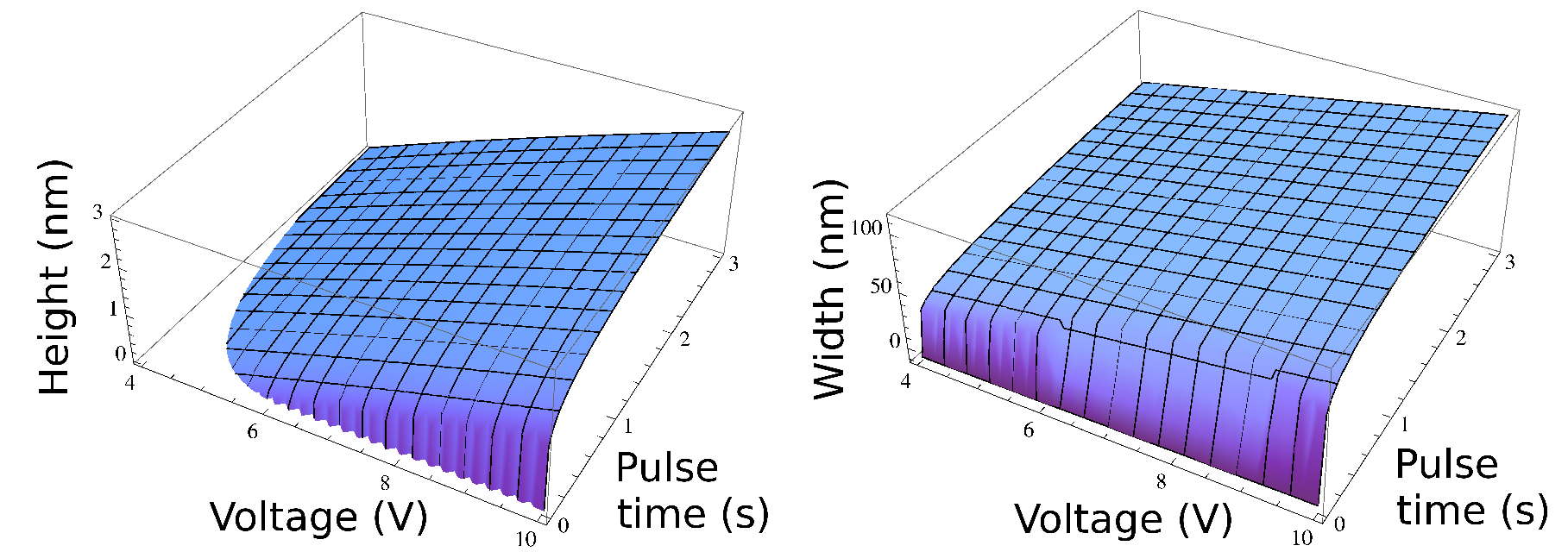In  the effect of humidity on the nanodot size is presented and the relationship is shown in Figure 3.16. A nearly linear relationship between humidity and the nanodot height is seen, while the width increases more rapidly, when the humidity is increased to 90%. This can be explained by the increase in water meniscus size with increased humidity, which provides more oxyanions to take part in the oxidation reaction.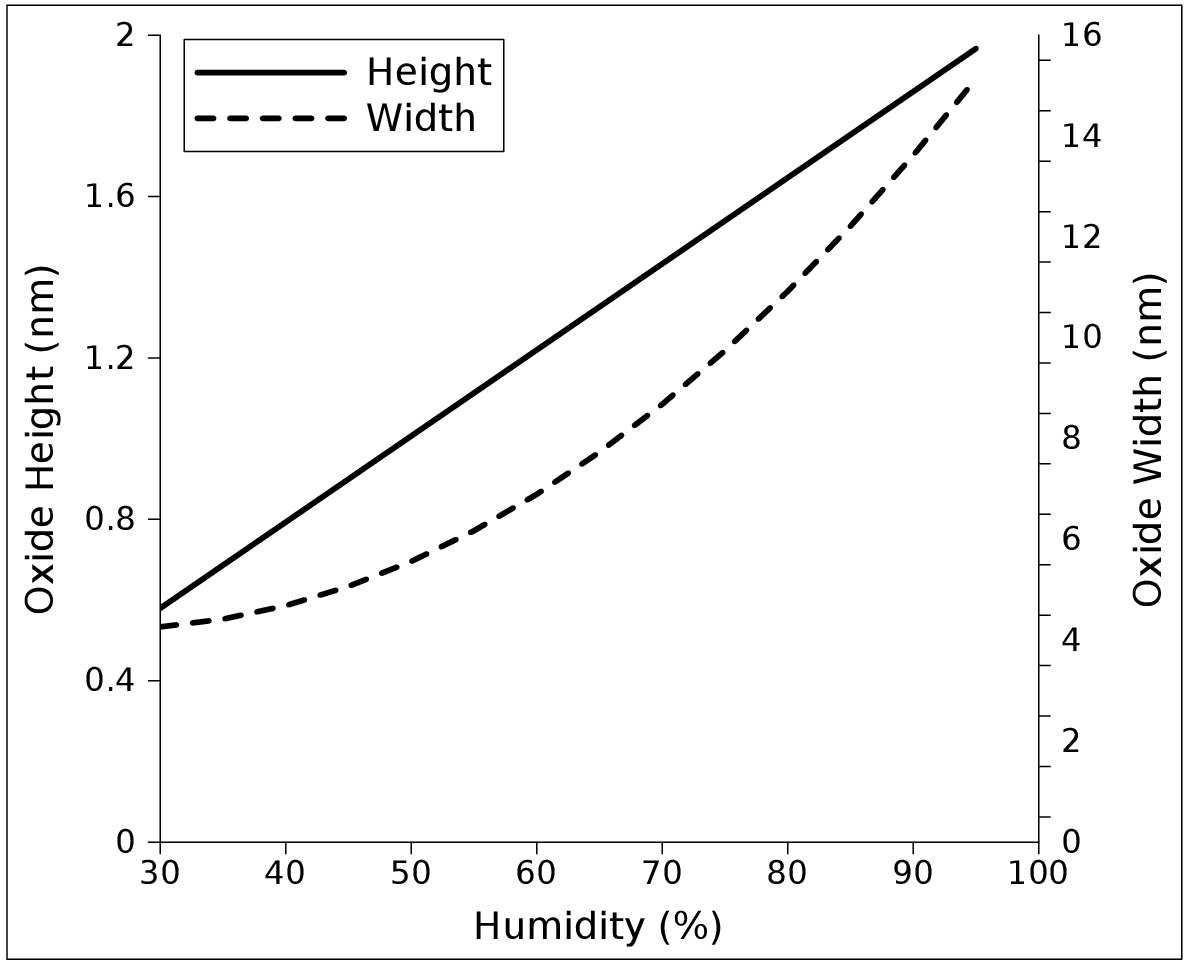The derived empirical equation which describes the effect of all three parameters (time, voltage, and humidity) on the height and width of the oxide dot in nanometers, produced using the AFM in NCM is given by: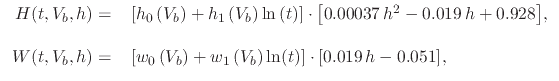(121)

whereis the pulse time in seconds,is the applied voltage in volts, andis the RAH in percent.

### 3.4.2.7 Model for nanowires generated in NCM

In addition to using experimental observations in order to implement an empirical model for nanodots in the LS simulator, the simulator has also been extended to include the generation of nanowires using an AFM in NCM. Although a nanowire can be generated using a sequence of nanodots placed such that the lateral distance between each dot is smaller than a dot's width, having a separate model for nanowires can help to speed up simulation times. Simulating a nanowire as a sequence of nanodots means that each nanodot needs its own simulation step, while having a single empirical equation which governs nanowire generation requires only one simulation step. From the experimental results found in , relevant information is extracted in order to include the effects on the nanowire shape due to variations in applied voltage, oxidation time, RAH, and wire orientation, shown in Figure 3.17. The wire orientation is represented as an angle, where the (010) direction is 0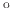while (100) is 90for a Si (100) sample, shown in Figure 3.18 and Table 3.1.

Table 3.1: Effects of wire orientation on the nanowire height and width, with=0.1ms,=7V, and=55%.
Orientation w.r.t. (010) Height (nm) FWHM (nm)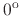1.07 38.3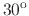1.93 65.1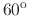1.30 83.4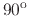1.19 62.8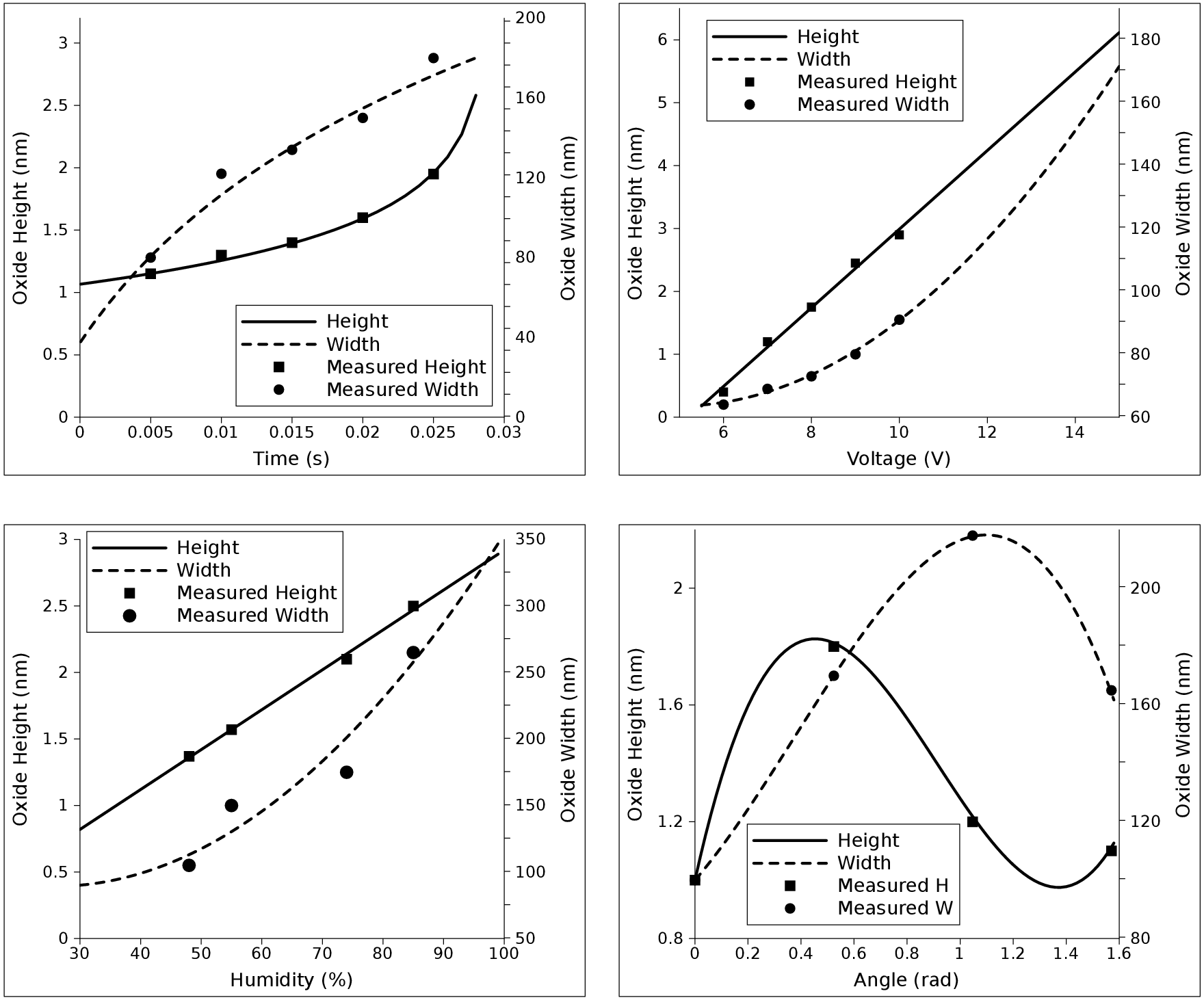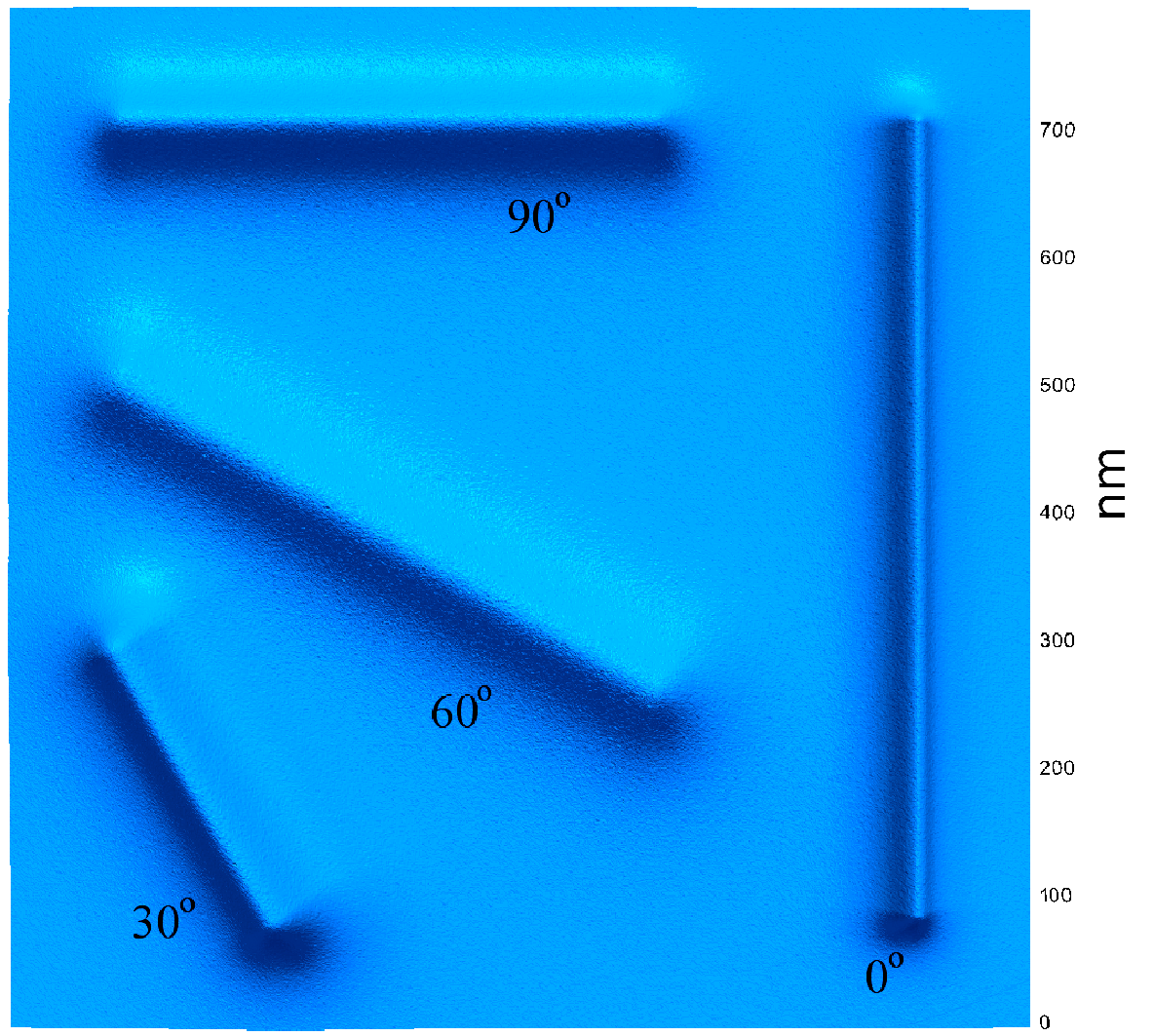It is evident that increasing the oxidation time, the applied voltage, or the RAH results in an increased nanowire height and width. However, the effect of the wire orientation is less predictable. The smallest nanowire is noted at an orientation of, while the largest nanowire height is noted atand the largest FWHM is noted at. The effect of the wire orientation on the height and width of the nanowire is not identical. The empirical equation, derived using the experimental results from  and implemented in the LS simulator is given by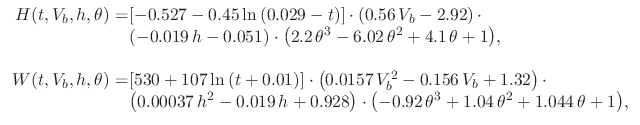(122)

whereis the oxidation time in seconds,is the applied voltage in volts,is the RAH in percent, andis the wire orientation in radians, represented as the angle between the nanowire and the (010) direction.

In Figure 3.17 the results of the empirical equation (3.51) generated for this work are compared to the experimental data from .

L. Filipovic: Topography Simulation of Novel Processing Techniques